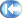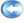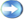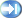Home|Add to Favorites|Add to My Toolkit International TradeHS of Japan|Register|Sign in|CustomizationHarmonized Tariff Schedule of the Japan  In Japan, The 9-digit statistical code consists of 6-digit HS code and 3-digit domestic codeďĽŚ are used for commodity classification in Customs declarations. Enter either the first part of an HTS category number up to 6 digits or any part of a product description.   ă€€
 6/14#nav{position:fixed; top:20px; left:30px;width:86px;height: 67px; border:1px solid #aaa;display: none;background: #fafafa;padding-left: 8px;padding-right: 8px;padding-top: 12px}
Harmonized Tariff Schedule
Statistical code Description
H.S. code Domestic code
5205.12 Measuring less than 714.29 decitex but not less than 232.56 decitex (exceeding 14 metric number but not exceeding 43 metric number)
5205.13 Measuring less than 232.56 decitex but not less than 192.31 decitex (exceeding 43 metric number but not exceeding 52 metric number)
5205.14 Measuring less than 192.31 decitex but not less than 125 decitex (exceeding 52 metric number but not exceeding 80 metric number)
5205.15 Measuring less than 125 decitex (exceeding 80 metric number)
5205.21 Measuring 714.29 decitex or more (not exceeding 14 metric number)
5205.22 Measuring less than 714.29 decitex but not less than 232.56 decitex (exceeding 14 metric number but not exceeding 43 metric number)
5205.23 Measuring less than 232.56 decitex but not less than 192.31 decitex (exceeding 43 metric number but not exceeding 52 metric number)
5205.24 Measuring less than 192.31 decitex but not less than 125 decitex (exceeding 52 metric number but not exceeding 80 metric number)
5205.26 Measuring less than 125 decitex but not less than 106.38 decitex (exceeding 80 metric number but not exceeding 94 metric number)
5205.27 Measuring less than 106.38 decitex but not less than 83.33 decitex (exceeding 94 metric number but not exceeding 120 metric number)
5205.28 Measuring less than 83.33 decitex (exceeding 120 metric number)
5205.31 Measuring per single yarn 714.29 decitex or more (not exceeding 14 metric number per single yarn)
5205.32 Measuring per single yarn less than 714.29 decitex but not less than 232.56 decitex (exceeding 14 metric number but not exceeding 43 metric number per single yarn)
5205.33 Measuring per single yarn less than 232.56 decitex but not less than 192.31 decitex (exceeding 43 metric number but not exceeding 52 metric number per single yarn)
5205.34 Measuring per single yarn less than 192.31 decitex but not less than 125 decitex (exceeding 52 metric number but not exceeding 80 metric number per single yarn)
5205.35 Measuring per single yarn less than 125 decitex (exceeding 80 metric number per single yarn)
5205.41 Measuring per single yarn 714.29 decitex or more (not exceeding 14 metric number per single yarn)
5205.42 Measuring per single yarn less than 714.29 decitex but not less than 232.56 decitex (exceeding 14 metric number but not exceeding 43 metric number per single yarn)
5205.43 Measuring per single yarn less than 232.56 decitex but not less than 192.31 decitex (exceeding 43 metric number but not exceeding 52 metric number per single yarn)
5205.44 Measuring per single yarn less than 192.31 decitex but not less than 125 decitex (exceeding 52 metric number but not exceeding 80 metric number per single yarn)
5205.46 Measuring per single yarn less than 125 decitex but not less than 106.38 decitex (exceeding 80 metric number but not exceeding 94 metric number per single yarn)
5205.47 Measuring per single yarn less than 106.38 decitex but not less than 83.33 decitex (exceeding 94 metric number but not exceeding 120 metric number per single yarn)
5205.48 Measuring per single yarn less than 83.33 decitex (exceeding 120 metric number per single yarn)

Top Use:Harmonized Tariff Schedule of Japans inquiry:  The

Recent user inquiry: